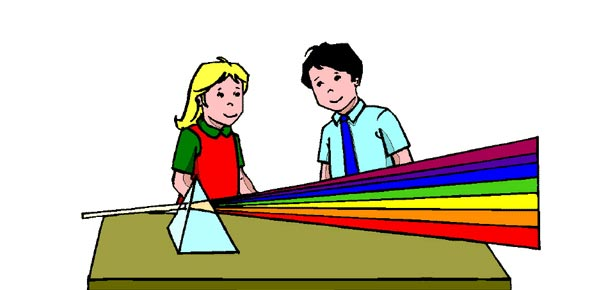# Chapter 26: Properties Of Light

10 Questions | Total Attempts: 1258Settings• 1.
Most of the waves in the electromagnetic spectrum are
• A.

Red light.

• B.

Blue light.

• C.

Green light.

• D.

Invisible.

• 2.
Electromagnetic waves consist of
• A.

Compressions and rarefactions of electromagnetic pulses.

• B.

Oscillating electric and magnetic fields.

• C.

Particles of light energy.

• D.

High-frequency gravitational waves.

• 3.
The fact that light travels at 300,000 km/s is a consequence of
• A.

Electromagnetic wave propagation.

• B.

Electromagnetic field induction.

• C.

Maxwell's laws.

• D.

Energy conservation.

• E.

None of these

• 4.
The source of all electromagnetic waves is
• A.

Changes in atomic energy levels.

• B.

Vibrating atoms.

• C.

Accelerating electric charges.

• D.

Crystalline fluctuations.

• E.

None of these

• 5.
The main difference between a radio wave and a light wave is its
• A.

Speed.

• B.

Wavelength.

• C.

Frequency.

• D.

All of these

• E.

Two of these

• 6.
Which of these electromagnetic waves has the shortest wavelength?
• A.

• B.

Infrared waves

• C.

X-rays

• D.

Ultraviolet waves

• E.

Light waves

• 7.
Compared to ultraviolet waves, the wavelength of infrared waves is
• A.

Shorter.

• B.

Longer.

• C.

The same.

• 8.
Compared to radio waves, the velocity of visible light waves in a vacuum is
• A.

Less.

• B.

More.

• C.

The same.

• 9.
If an electric charge is shaken up and down
• A.

Sound is emitted.

• B.

Light is emitted.

• C.

Electron excitation occurs.

• D.

A magnetic field is created

• E.

Its mass decreases.

• 10.
If an electron vibrates up and down 1000 times each second, it generates an electromagnetic wave having a
• A.

Period of 1000 s.

• B.

Speed of 1000 m/s.

• C.

Wavelength of 1000 m.

• D.

Frequency of 1000 Hz.

• E.

Wavelength of 1000 km.

Related Topics